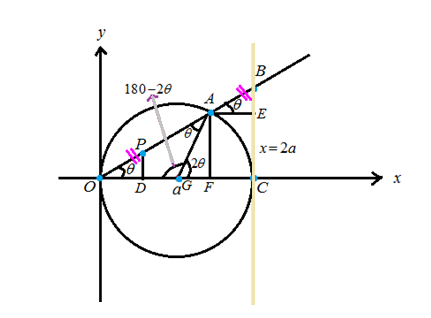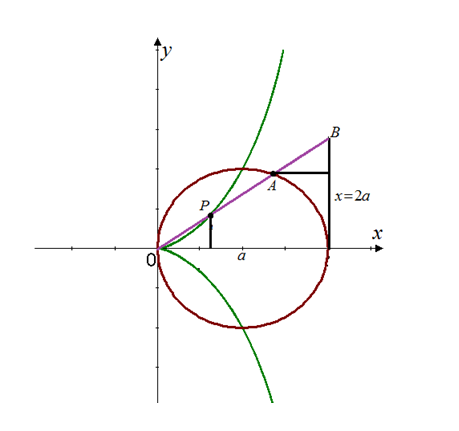# Find parametric equations for the set of all points P .### Single Variable Calculus: Concepts...

4th Edition
James Stewart
Publisher: Cengage Learning
ISBN: 9781337687805### Single Variable Calculus: Concepts...

4th Edition
James Stewart
Publisher: Cengage Learning
ISBN: 9781337687805

#### Solutions

Chapter 1, Problem 34RE

a.

To determine

## Find parametric equations for the set of all points P .

Expert Solution

(aacos2θ,2atanθasin2θ)

### Explanation of Solution

Given information:

Find parametric equations for the set of all points P determined as shown in the figure such that |OP|=|AB| .(This curve is called the cissoid of Diocles after the Greek scholar Diocles, who introduced the cissode as a graphical method for constructing the edge of a cube whose volume is twice that of a given cube.)Calculation:

Consider the figureWhereas OG&GA are radii of the circle radius a . So OG&GA are congruent and so their opposite angles OAG&AOG are congruent.

Hence OAG=AOG=θ .

For the triangles ABE&ODP , the sides |OP|=|AB| and lines BEPD (these lines are perpendicular to the xaxis ) so the angles BAE&POD are congruent.

Hence BAE=POD=θ .

Consider the triangle OBC to find the coordinates of the point B .

Hence OC=2a (diameter of the circle) and BOC=θ , we have

tanθ=BC2aBC=2atanθ

The x -coordinate of the point B is 2a and the y coordinates is 2atanθ .

Hence the point B=(2a,2atanθ) .

To find the point A , we need to determine the distances AF&OF .

In the triangle OAG , sum of the angles are

θ+θ+AGO=180°AGO=180°2θ

We know that

AGO+AGF=180°180°2θ+AGF=180°AGF=2θ

Now consider the triangle AGF, get

sin2θ=AFAG=AFaAF=asin2θ

And

cos2θ=GFAG=GFaGF=acos2θ

The x coordinate of the point A is

OG+GF=a+acos2θ

The y coordinate of the point A is AF=asin2θ .

Hence, the point A=(a+cos2θ,asin2θ) .

Find the distance AE&BE:

To get the distance AE , we need to subtract the x -coordinate of A from the x -coordinate of B .

AE=2a(a+acos2θ)=aacos2θ

To get the distance BE , we need to subtract the y -coordinate of A from the y -coordinate of B .

BE=2atanθasin2θ .

The triangles ABE&OPD are congruent whereas the sides OP,AB are equal and the angles BAE&POD are equal.

Hence, in these two triangles ABE&OPD the corresponding sides AE,OD are equal and BE,PD are equal.

AE=OD=aacos2θ,BE=PD=2atanθasin2θ

Hence, the required coordinates of the point P is (aacos2θ,2atanθasin2θ) .

b.

To determine

### Use the geometric description of the curve to draw a rough of the curve by hand.

Expert Solution### Explanation of Solution

Given information:

Use the geometric description of the curve to draw a rough of the curve by hand. Check your work by using the parametric equations to graph the curve.Calculation:

Draw the curve represented by the parametric equations

x(θ)=aacos2θ,y(θ)=2atanθasin2θ .

This curve is known as cissoids of Diocles.

The graph of the curve is shown below:Hence, the result is plotted.

### Have a homework question?

Subscribe to bartleby learn! Ask subject matter experts 30 homework questions each month. Plus, you’ll have access to millions of step-by-step textbook answers!